## 3.4 Notation

There exist several ways to write the derivations done with natural deduction. I will use the Fitch style, because it's the one I used when learning, it's easy to understand, and occupies little space. It's something like this: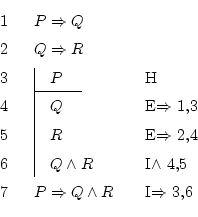This is sufficient to prove the validity of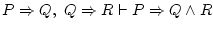.

That figure is to be done line by line, from top to the bottom. The numbers from the left show the number of each line, and are always in natural order.

The first lines contain each of the formulas which are written in the left part of the sequent. In this case, they are two: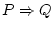and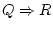. From these we will have to achieve the formula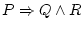.

On each line we write what new thing we have just discovered to be true, and to the right we note how did we discover that. Those symbols from the right side (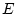and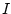) are the abbreviations of the names of the 9 rules. For example, here we can see implication elimination (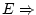), conjunction introduction (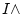), and implication introduction (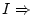). The numbers that go with them give us information about from where did we extract each necessary formula which is needed to apply the rule. They are line numbers, so, to be able to apply a rule, one has to use information only from the lines already written.

Finally, that vertical line which goes from line 3 to 6 it's a hypothesis (that's why we putto the right). Everything which is inside it, is not always true, but only when happens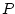(the heading of the hypothesis, at line 3). So, all of the work we do inside the hypothesis cannot be used outside it, because it can't be assured to be always true.

The procedure finishes when we discover that it's true the formula at the right side of the sequent, in this case(it appears at the last line).

Daniel Clemente Laboreo 2005-05-17